# RBSE Solutions For Class 12 Physics Chapter 16: Electronics | Textbook Important Questions & Answers

The answers are provided for all the questions of Chapter 16 Physics of RBSE Class 12. Students can go through these questions to understand the concepts better and score well in the board examination and entrance examinations for various professional courses. These solutions are provided by the team of experts to provide the best and accurate solutions to the questions. Do check BYJU’S RBSE Class 12 solutions page to get the answers for textbook questions of all subjects.

## Multiple Choice Questions

Q1: At absolute zero temperature intrinsic Germanian and intrinsic Siliconeare

1. Superconductors
2. Good semiconductors
3. Ideal insulator
4. Conductors

Q2: In insulators the forbidden energy gap between the valence band and conduction band is

1. 1 eV
2. 0.6 eV
3. 0.1 eV
4. 0.01 eV

Q3: If an NPN transistor is used as an amplifier then

1. Electrons move from base to collector
2. Holes move from emitter to base
3. Holes move from base to emitter
4. Electrons move from emitter to base

Q4: The current amplification factor for a common base circuit of a transistor is 0.95. When emitter current is 1mA then base current is

1. 0.1 mA
2. 0.2 mA
3. 0.19 mA
4. 1.9 mA

Q5: The forbidden energy gap in Germanium is 0.7 eV. The wavelength at which its absorption starts is

1. 35000 Å
2. 17700 Å
3. 25000 Å
4. 51600 Å

## Very Short Answer Type Questions

Q1. What is the direction of diffusion current in junction diode?

Answer: The direction of current is from P region to N region.

Q2: Write down the relation between α and β (current amplification factor) of the transistor.

Answer: The relation between α and β is given by

β =α/(1-α)

Or α = β/(β+1)

Q3: Can the barrier potential of the forward-biased PN junction be measured by a voltmeter connected at the ends of the device?

Answer: No, it is not possible to find the barrier potential of the forward-biased PN junction by connecting voltmeter at the ends.

Q4: Make the truth table for the OR gate.

 A B Y =A+B 0 0 0 1 0 1 0 1 1 1 1 1

Q5: Write down the name of that logic gate in which the output is 1 when all the inputs are 1

Answer: AND gate gives the output 1 when the input is 1

Q6: To use the transistor as an amplifier which junction is reverse biased?

Answer: To use the transistor as an amplifier Base-collector junction is reverse biased.

Q7: What will be the value of α for a transistor for which β= 19?

Answer: The relation between α and β is given by

α = β/(β+1) = 19/(19+1) = 19/20 = 0.95

Q1: What is rectification? Draw the figure of a bridge full-wave rectifier.

Rectification: It is the process of converting alternating current to direct current.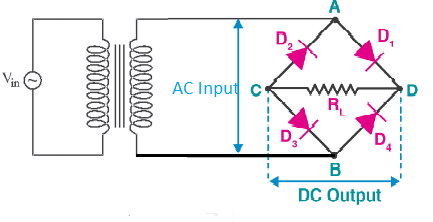Q2: In a rectifier why is the base made thin in comparison to the emitter and collector?

Answer: Let us understand this by considering an NPN transistor. The injected electrons from the emitter should pass through the base region to reach the collector. The injected electrons from the emitter recombine with the holes in the base. When they recombine the injected electrons are destroyed. To keep this recombination in the base region minimum it is kept very thin. 98％ of the injected electrons should reach the collector.

Q3: Make complete I-V characteristics curve for ideal PN junction diode. Define dynamic resistance in the forward-biased state.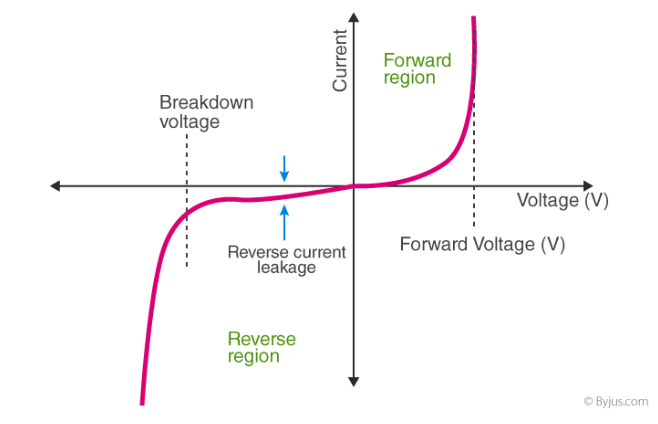Dynamic resistance of a diode is defined as the ratio of change in voltage to the change in current.

Q4: What do you understand by the logic gate? Draw the symbol for XOR gate and also write the truth table.

Answer: A Logic gate is an elementary building block of a digital circuit. The logic gates have two or more inputs and a single output. It follows a certain logical relationship between the input and the output voltages. The five common logic gates are OR gate, AND gate, NOT gate, NOR gate and NAND gate. Each logic gate has a symbol and its function is defined by the truth table.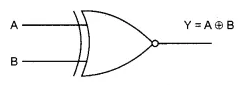The Boolean expression for XOR gate is Y=A ⊕ B. If any one of the inputs A or B has the value 1 then the output Y = 1. If both the inputs are 0 or 1 then the output is 0. Hence the truth table for XOR gate is

 A B Y =A ⊕ B 0 0 0 1 0 1 0 1 1 1 1 0

Q5: Draw the transistor-based circuit diagram for NOT gate and also give its truth table.

Transistor-based circuit diagram for NOT gate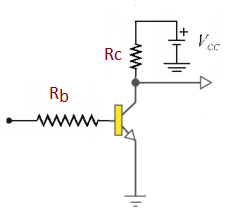The Boolean expression for NOT gate is given by Y = Ā. If the input is A=0 then Y = Ā = 1 and vice versa.

 A Y = Ā 0 1 1 0

Q6: Write down the boolean expression for the given logic circuit. And also write the truth table for it.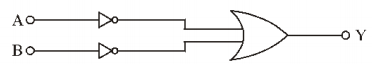Answer: In the above logic gate the output of the two NOT gate is given as the input in the OR gate. The Boolean expression for NOT gate is given by Y = Ā. If the input is A=0 then Y = Ā = 1 and vice versa. The Boolean expression for OR gate is given by Y = A+B. The output of the OR gate is 1 if either one of the input is 1.

 Input Output of NOT gate Final output of OR gate A B Y1 = A Y2= B Y = A+B 0 0 1 1 1 0 1 1 0 1 1 0 0 1 1 1 1 0 0 0

Q7: Draw the circuit used for voltage regulation by Zener diode and also explain the process in short.

Answer: The Zener diode is specially designed to work in reverse biased condition. These diodes have specified breakdown voltage depending on the doping levels. As the voltage across the diode remains constant at the breakdown and there is an increase in current. This property helps Zener diodes to be used in voltage stabilization and voltage regulation.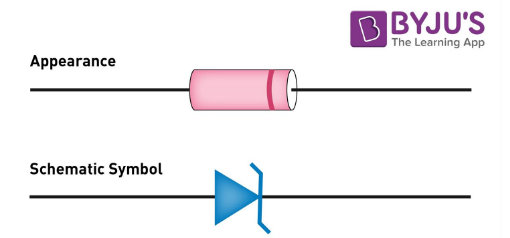##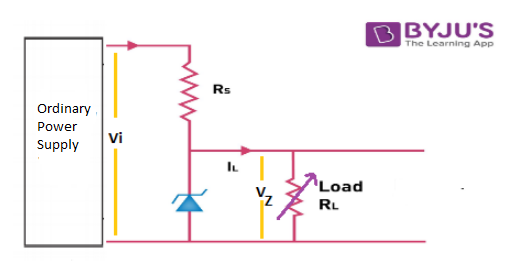The above figure shows a power supply with output voltage Vi, resistance Rs and Zener diode are connected across the output terminals. The load resistor RL is connected parallel to the Zener diode. The Zener diode selected has a breakdown voltage Vz equal to the constant value of the dc voltage to be obtained at the load resistance RL. The Zener diode is connected in the circuit in reverse biased condition. We do not use ordinary junction diode because the low power rating diode can get damaged when we apply reverse bias above its breakdown voltage. If the value of Vi is greater than VZ then the diode comes in the breakdown region and the potential across its terminals will be VZ. Since RL is parallel to the diode the potential difference across RL is also VZ. Even if the value of RL is changed the potential difference will be VZ. The series resistor Rs is connected to the circuit in order to limit the current into the diode.

## Essay Type Questions

Q1: Differentiate between conductors, insulators and semiconductors on the basis of energy band theory. Explain the process of electrical conductance in intrinsic semiconductors.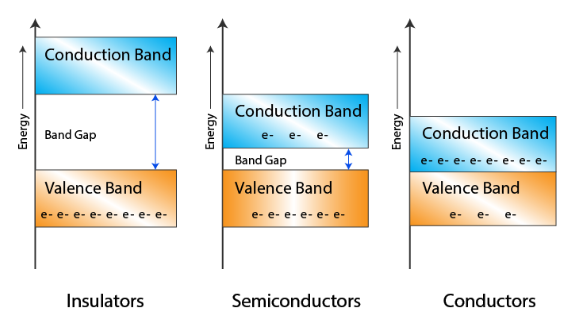Conductors

Metals like Gold, Copper, Aluminum, Silver, allow an electric current to flow through them.

The valence band and the conduction band overlap since there is no forbidden gap between them. The number of free electrons available in a conductor at room temperature is large.

Insulators

Insulators do not allow electricity to pass through them. The resistivity of a conductor is high and the conductivity value is low. The forbidden energy gap between the valence band and the conduction band in the insulator is very high up to 7eV. The movement of the electrons from the valence band to the conduction band is not possible because of the gap.Glass and wood are examples of the insulator.

Semiconductors

The energy band diagram of a semiconductor shows that the conduction band is empty and the valence band is completely filled. The forbidden gap between the two bands is very small, that is about 1eV approximately. For Germanium, the forbidden gap is 0.72eV and for Silicon, it is 1.1eV. Thus, a semiconductor requires small conductivity. Germanium and Silicon are the most preferable materials used as semiconductors.

Intrinsic Semiconductors

Semiconductors that are chemically pure, in other words, free from impurities are termed as intrinsic semiconductors. The number of holes and electrons is therefore determined by the properties of the material itself instead of the impurities. In intrinsic semiconductors, the number of excited electrons is equal to the number of holes; n = p. They are also termed as undoped semiconductors or i-type semiconductors. Silicon and germanium are examples of i-type semiconductors.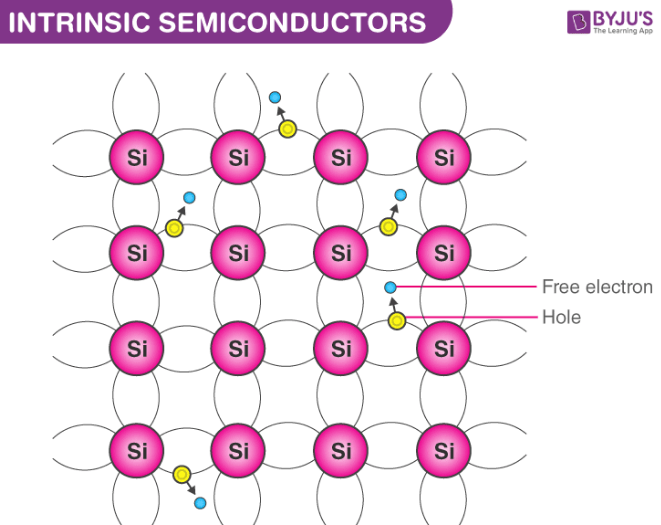Electronic Configuration of Silicon and Germanium

Silicon 1s2 2s22p6 3s2 3p2

Germanium 1s2 2s2 2p6 3s2 3p6 4s2 3d10 4p

We notice from the electron configurations of both the elements that they have four electrons in their outermost shell or valence shell. As the temperature of the semiconductor is increased, the electrons gain more thermal energy and thus break free from their shell. The process of ionization of the atoms in the crystal lattice creates a vacancy in the bond between the atoms. The position from which the electron gets dislodged has a hole which is equivalent to an effective positive charge. The hole is then occupied by a free electron, as a result of which the latter vacant position becomes a hole and the former becomes a neutral position. This way the hole or the effective positive charge is transferred from one position to another. In an intrinsic semiconductor, the number of free electrons is equal to the number of holes. Mathematically,

ne = nh =ni

Here, the ni gives the number of total intrinsic carrier concentration which is equal to the total number of holes or the total number of electrons.

When the temperature of an intrinsic semiconductor is T=0K, it behaves like an insulator. When the temperature is increased further, (T>0), the electrons get excited and move from the valence band to the conduction band. These electrons occupy the conduction band partially, leaving a correspondingly equal number of holes in the valence band.

Q2: What is PN junction? Explain the process occurring during its formation on the junction surface. If this junction is forward biased then explain the effect on the depletion layer.

A p-n junction is an interface or a boundary between two semiconductor material types, namely the p-type and the n-type. The p-side of the semiconductor has an excess of holes and the n-side has an excess of electrons.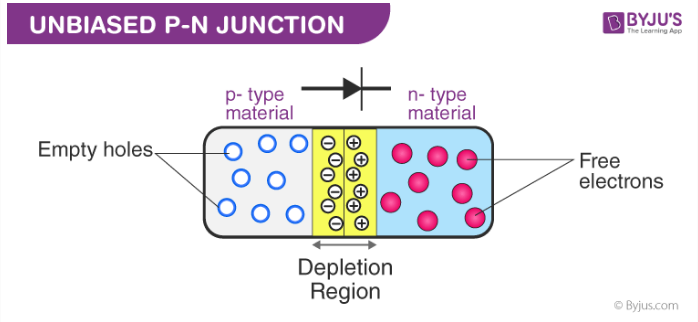After the p-n junction is formed a process called diffusion and drift occurs at the junction The concentration of electrons and holes differs on either side of the junction. As a result of which the holes from the p-side diffuse to the n-side and the electrons from the n-side diffuse to the p-side. This results in diffusion current across the junction.

Also when the electrons diffuse an ionised donor is left behind at the n-side. As this process continues a positively charged layer is developed on the n-side of the junctions. Similarly, an ionized acceptor is formed on the p-side when a hole moves from p-side to n-side. This region of positive charge and negative charge on either side of the junction is termed as the depletion region.

Due to this positive charge and negative charge region on either side of the junction, an electric field direction from positive charge towards the negative charge is developed. Due to this electric field, an electron on the p-side of the junction moves to the n-side of the junction. This motion is called drift. Here, we see that the direction of drift current is opposite to that of the diffusion current.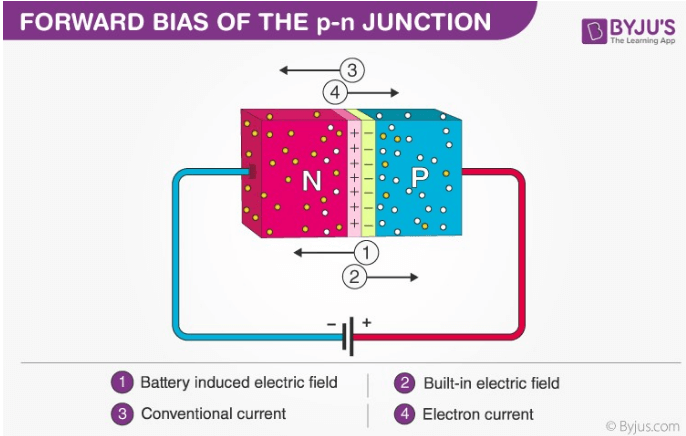The p-n junction diode is forward biased when the n-side is connected to the negative terminal of the battery and p- side is connected to the positive side. The applied electric field is opposite in direction to the inbuilt electric field in the p-n junction. The resultant electric field has a magnitude less than the built-in electric field. This results in a thinner depletion region which is less resistive. When the applied voltage is large then the depletion region’s resistance becomes negligible. In silicon, at the voltage of 0.6 V, the resistance of the depletion region becomes completely negligible and the current flows across it unimpeded.

Q3: What is a junction transistor? Make the necessary diagram and explain the working of a PNP transistor.

Answer: Junction transistor is a very important device in which there are two p-n junctions. These are of two types NPN and PNP. The middle part (base) is thin and highly doped. The other two parts are emitter and collector. The emitter has higher doping than the collector.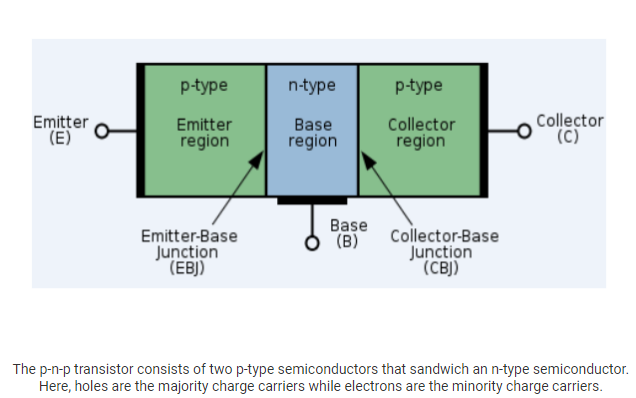Working of a PNP transistor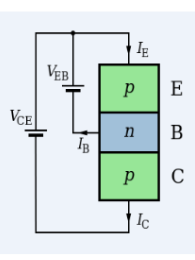The emitter-base junction is forward biased whereas the collector-base junction is reverse biased. In a PNP transistor, the majority charge carriers are holes which move from the emitter to the base. As the base layer is very thin, only little interaction occurs when electrons and holes combine. Most of the holes reach the collector. The current is carried by holes in a PNP transistor.